# Particle Dynamics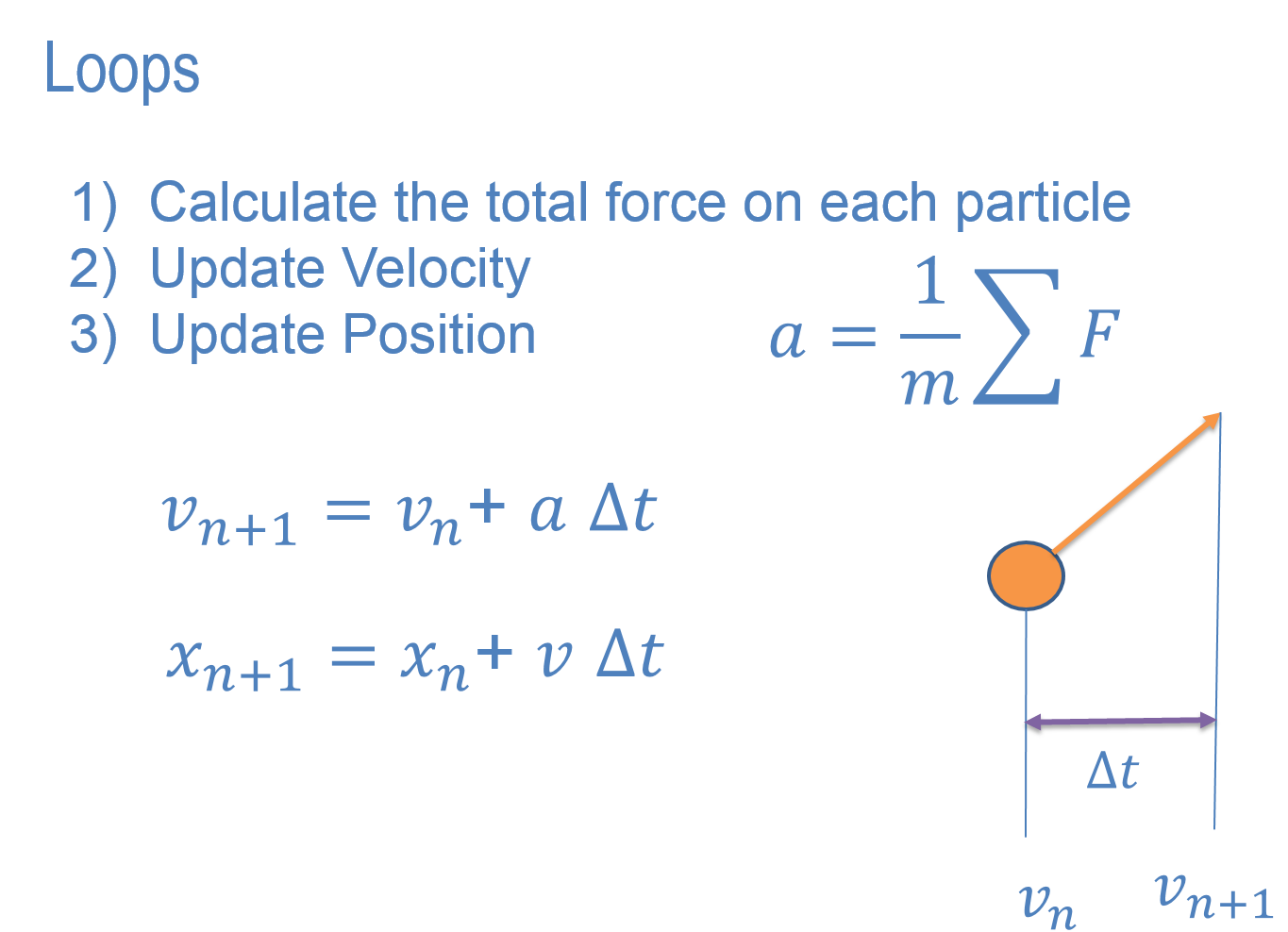## Newton's Equations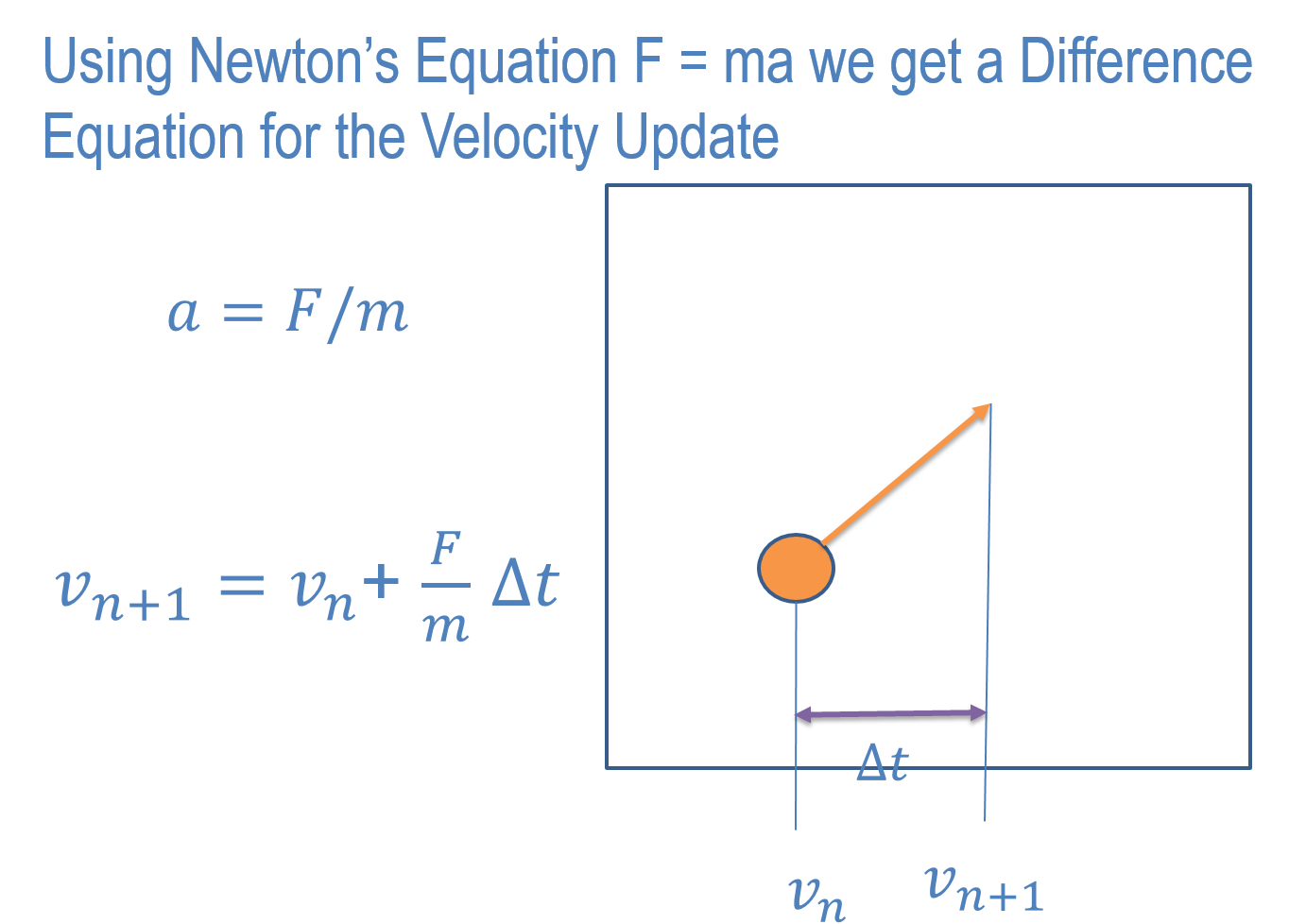## Newton's Equations## Newton's Equations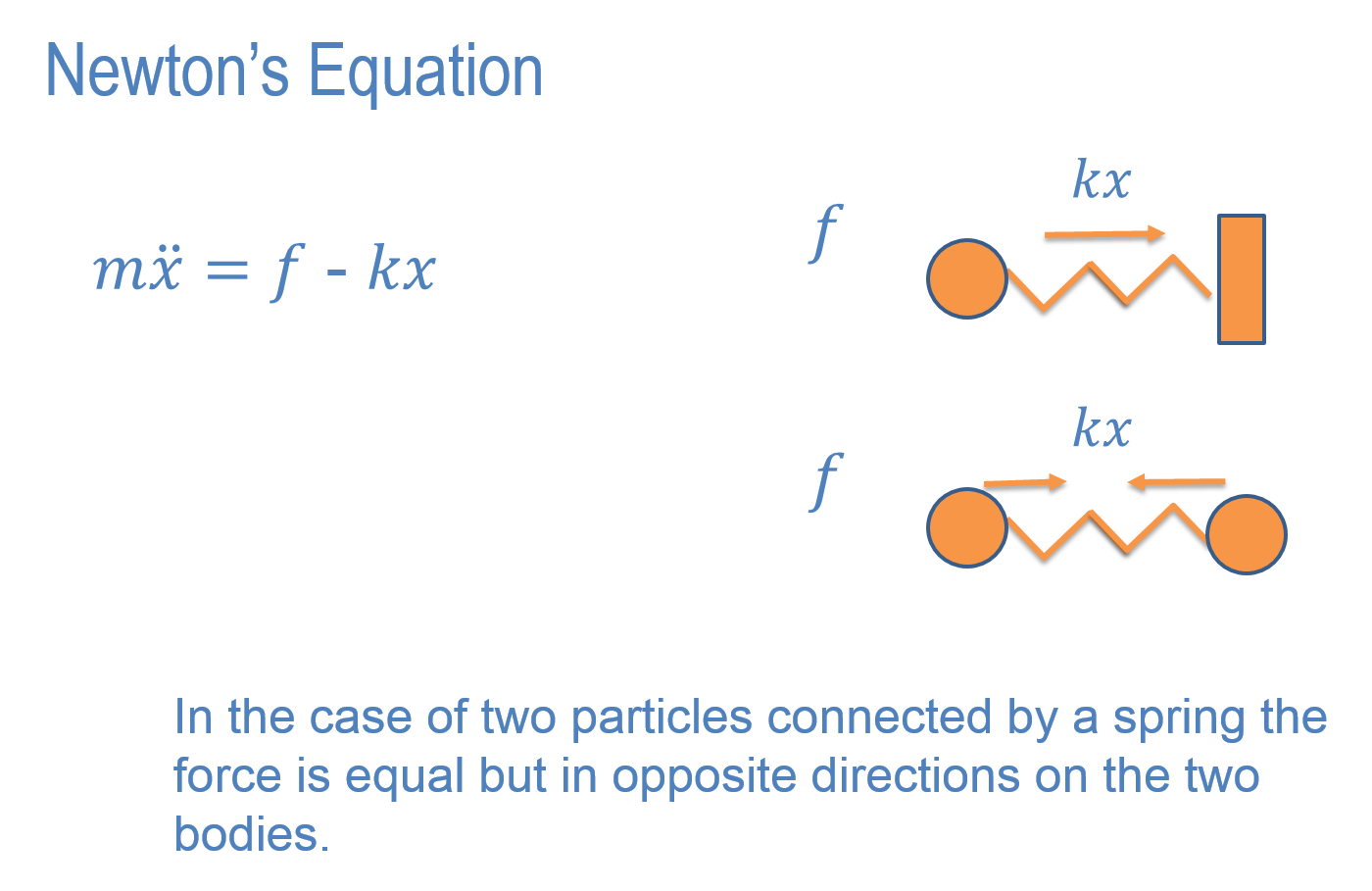## Newton's Equations## Newton's Equations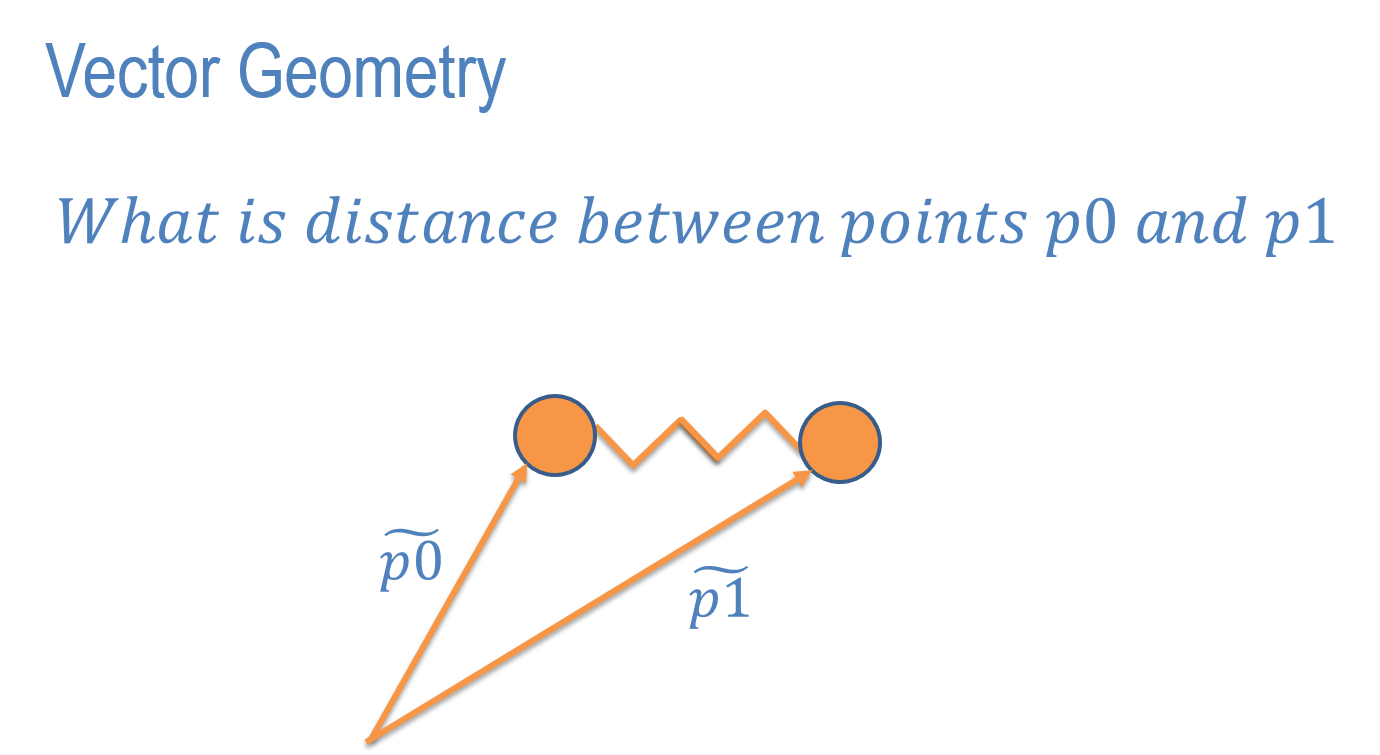## Newton's Equations## Newton's Equations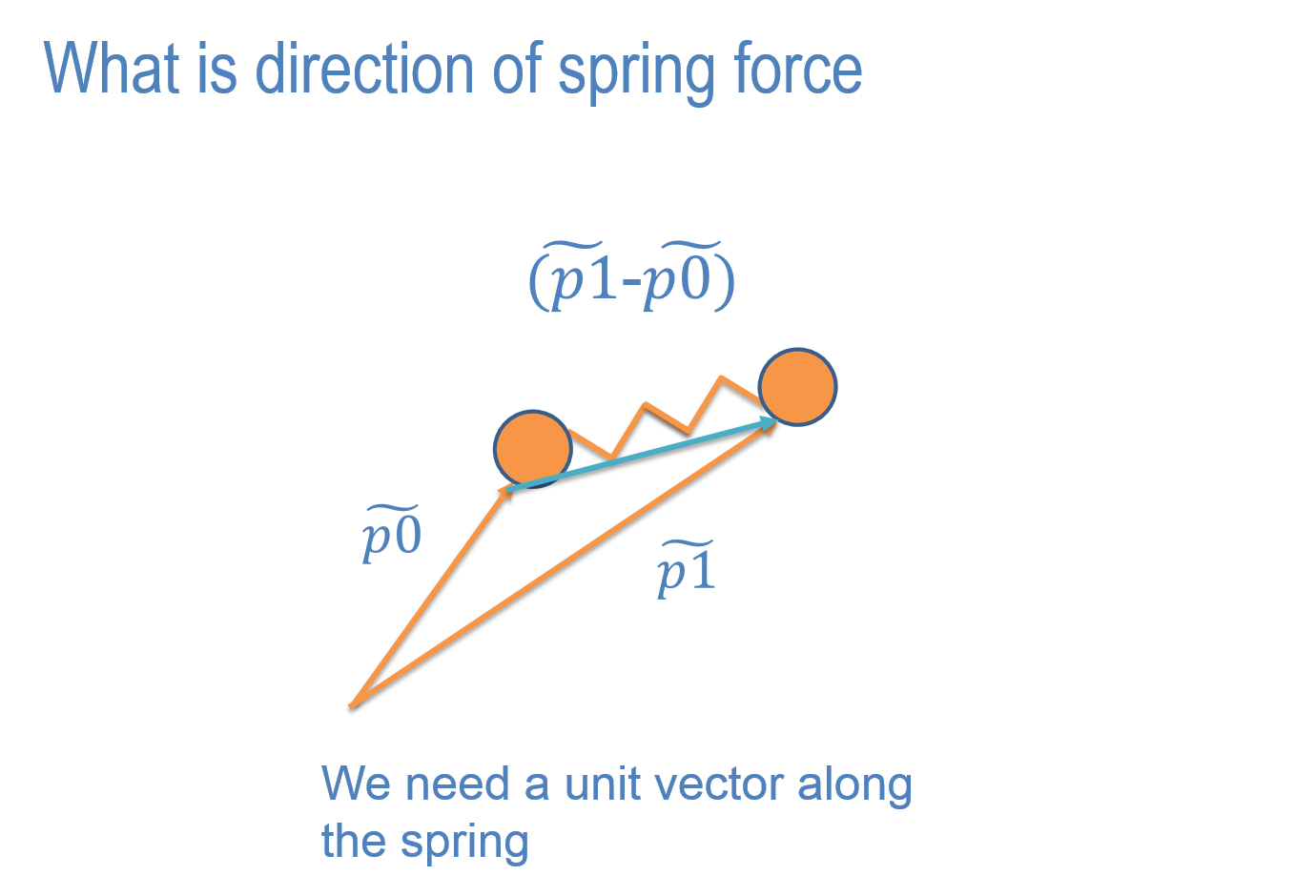In class Assignment 1.
Here is code starter code to create masses and springs. We'll start with just two masses and one spring to test the code.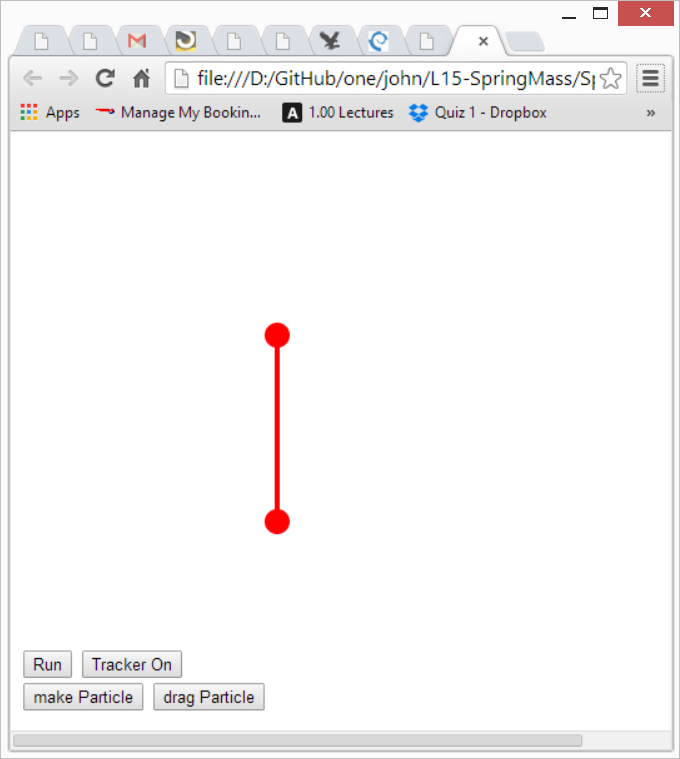Create two particles. Drag the fixed one so that you get the other to oscillate and create at least two different patters for the orbits. Hand in your screen shots.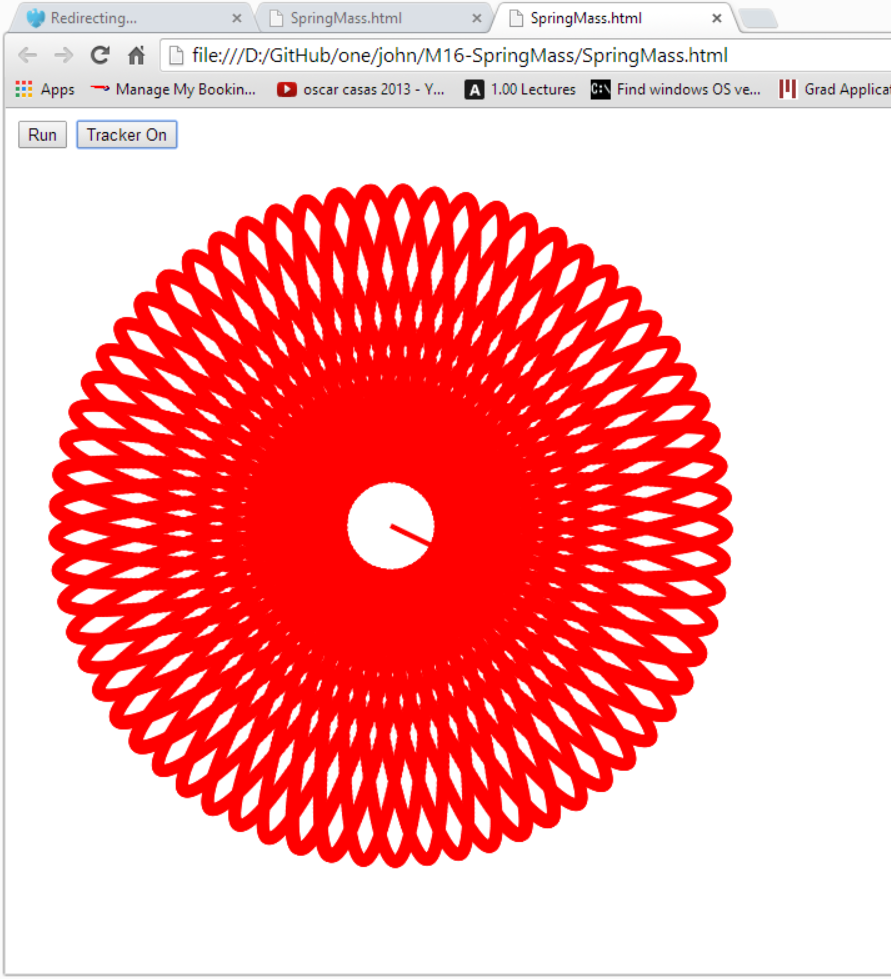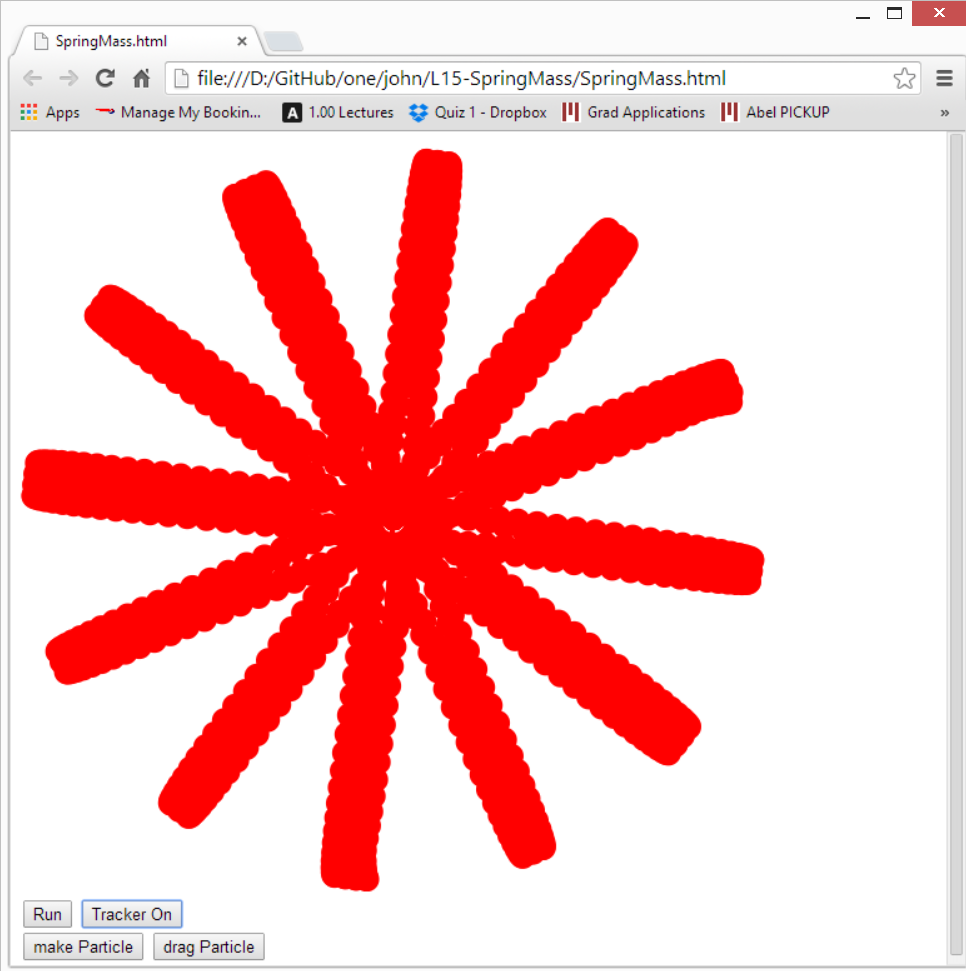Assignment 2
Change the code so that 3 particles are tied together by 2 springs. Hand in a screen shot of the two particles oscillating about the fixed one.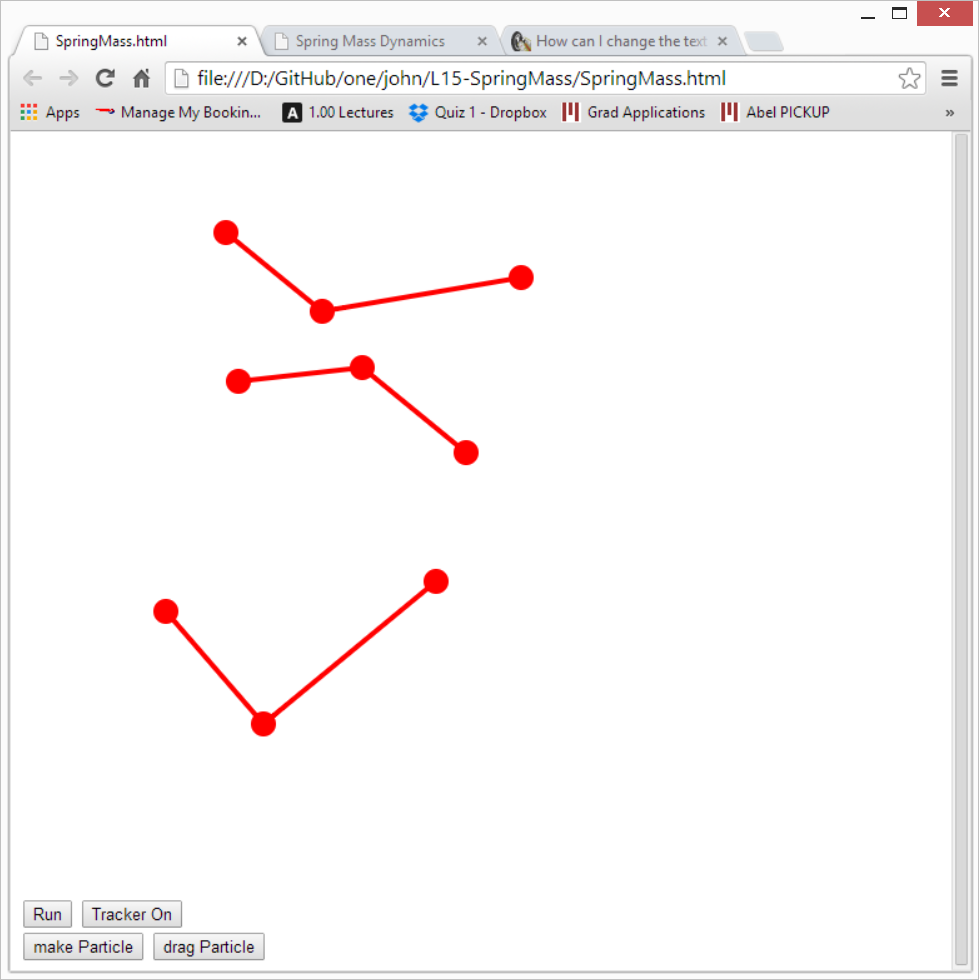Assignment 3 - Fracture of Materials
If the force in any of the springs exceeds 20 then remove that spring. ie the particles break apart. Make at least 3 groups of 3 particles per group and hand in a screen shot after two of the groups break apart. Hint: Find the code where the spring force is generated. Flag the spring if its greater than 20 (use setDeleteSpring = 'this' to grab a reference to the spring object.) Now after the loop over all springs use var k = springs.indexOf(setDeleteSpring) and then delete the spring from 'springs' by using 'splice'